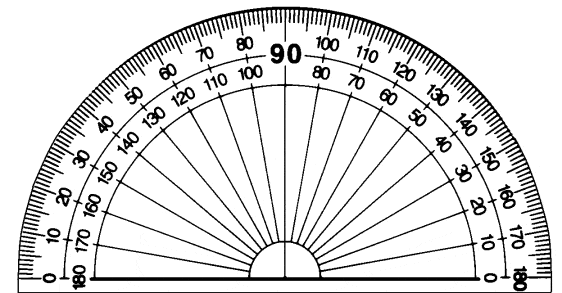# Problem involving moment components and angles

1
Gripper C of the industrial robot is accidentally subjected to a 60 lb side load directed perpendicular to BC (see attachment). The lengths of the robot's links are AB = 22 in and BC = 18 in. By using the moment components method, determine the moment of the force about the center of joint A.

2
M = Fd (d is the lever arm)

3
I'm not sure what angle to use when calculating the components of the 60 lb force. Is the 60 lb force at a 140° angle with the coordinate plane given at point A? Since this is the first step of the problem, I want to make sure I'm doing it correctly.

#### Attachments

•20130924_160837.jpg
23.1 KB · Views: 836
Last edited:

SteamKing
Staff Emeritus
Homework Helper
I don't know where you get 140 deg. from. Most right angles are 90 deg., and BC is 55 deg. above the horizontal.

The 140 degrees was referring to the direction of the force (in relation to the xy coordinate frame thats given at point A). I don't know which angles to use to calculate the force components. Would I use 55 degrees?

SteamKing
Staff Emeritus
Homework Helper
You want the moment calculated about A in component form. I suggest you find out what the components of the 60-lb Force are relative to the x-y axis. You know what angle BC makes with the x-axis, and the force is acting a right angles to BC.

So the correct angle would be:

180-90-55 = 35 degrees?

I think I may have just over thought this...

SteamKing
Staff Emeritus
Homework Helper
Take a look at the force vector. Look at the position of the arrow head relative to the opposite end of the vector. What quadrant is the arrow head in. Is that the same quadrant that an angle of 35 degrees would be in?

If these things are confusing, you can always use a protractor to check yourself. They are handy tools for this sort of work.35 should be the reference angle, which means that 145 would be the real angle, since Fx needs to be negative. Right?

SteamKing
Staff Emeritus
•